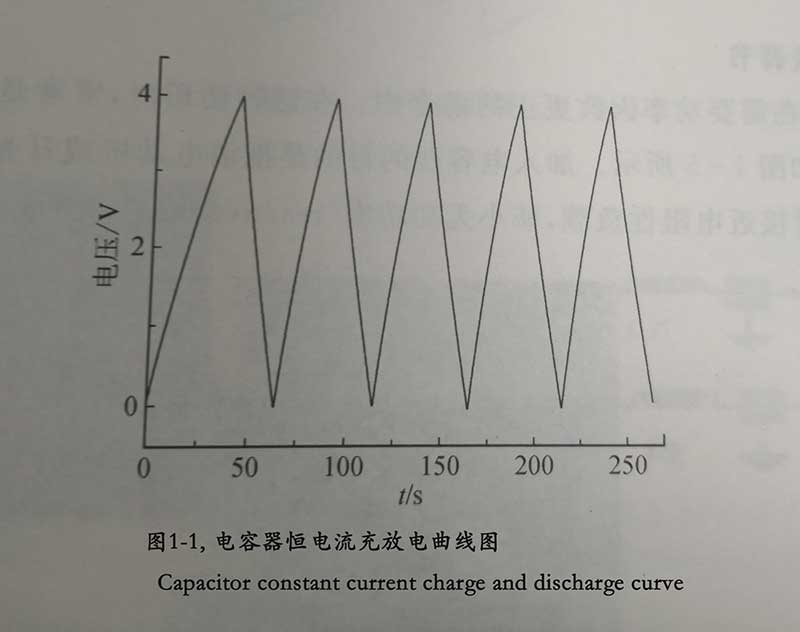Capacitor circuit characteristics

👉Traditional dielectric capacitors, supercapacitors and chemical batteries are all energy storage electronic devices, all of which have the function of storing electrical energy. The charge on the capacitor is the integral of the charging current “i” to the time “t”, as shown in the following formula:

🌞Q=∫idt (Formula 1)

According to the formula, C=Q/U, when the capacitance of the capacitor is constant, the following formula holds:

🌞Dq=CdU (Formula 2)

Substituting formula 1 into formula 2 can be obtained:

🌞DU/dt=i/C (Formula 3)

👉It can be seen from Formula 3 that when the capacitor is charged with a constant current, the voltage across the capacitor will increase linearly with time; on the contrary, when the current device is discharged with a constant circuit, the voltage across the capacitor will decrease linearly with time, and its slope is the same as the capacitance. It is inversely proportional, as shown in Figure (1-1) (Because the capacitor has a certain leakage current, the charging and discharging curve slightly deviates from the linearity during charging and discharging)

Capacitor constant current charge and discharge curveCapacitor constant current charge and discharge curve
👉From the constant current charge and discharge curve of the capacitor in Figure 1-1, it can be seen that the constant current charge and discharge curve of the capacitor is a sawtooth waveform, which is fundamentally different from the constant current charge and discharge curve of the battery. The charge and discharge curve of the battery has a charge or Put a point on the voltage platform. Supercapacitors have a sawtooth waveform constant current charging and discharging curve, which is a “true capacitance” rather than a “pseudocapacitance”.Capacitor circuit characteristics

👉When the charging current of the capacitor is i and the charged voltage reaches U, the energy stored in the capacitor is:

🌞W=∫iUdt = ∫C(dU)/(dt)Udt = C∫UdU = 1/2*CU2.